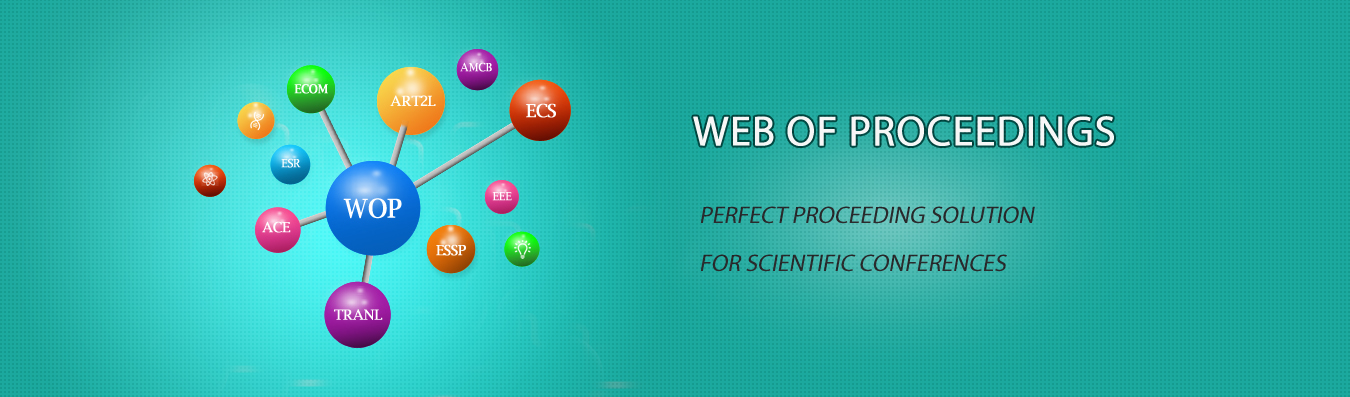The best way to conference proceedings by Francis Academic Press### The Application of Mathematical Experiment in the Teaching of College Mathematics

DOI: 10.25236/iwedss.2019.321

#### Author(s)

Li Xingjun, Cheng Hongping

Li Xingjun

#### Abstract

Mathematical experiment is an interesting course, requiring computer and mathematical logic. It focuses on the practice and thinking of college students, so as to increase its interestingness and students’ enthusiasm for learning. College students will be faced with a large number of calculations, however, by virtue of mathematical experiment, mathematical model can be built through computer technology, and students can get rid of complex and boring mathematical calculations. The combination of teaching and computer technology can make it easier for students to learn college mathematics and reduce their calculation pressure, at the same time, students will prefer the course of college mathematics.

#### Keywords

Mathematical experiment, college mathematics, teaching